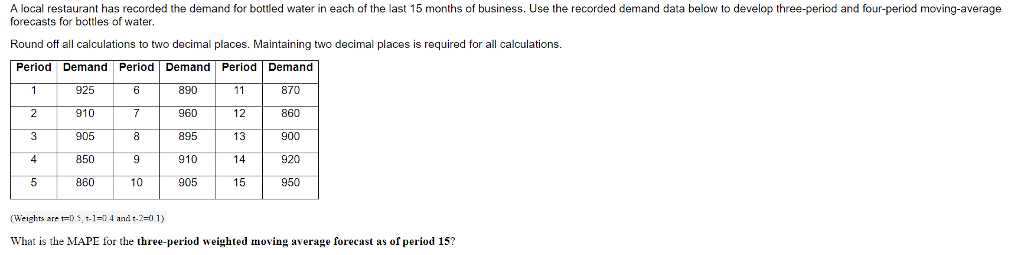# Question & Answer: A local restaurant has recorded the demand for bottled water in each of the last…..

(Weights are t=0.3, t-1=0.2, t-2=0.3 and t-3=0.2)A local restaurant has recorded the demand for bottled water in each of the last 15 months of business. Use the recorded demand data below to develop three-period and four-period moving-average forecasts for bottles of water. Round off all calculations to two decimal places. Maintaining two decimal places is required for all calculations. (Weights are t = t-1 = 0.4 and t-2 = 0.1) What is the MAPE for the three-period weighted moving average forecast as of period 15?

Please see relevant data on three period moving average , 4 period moving average and MAPE for 3 period moving average as presented in below table :

 Period Demand Forecast -Three period moving average Absolute Percentage error Forecast – Four Period Moving Average 1 925 2 910 3 905 4 850 917 7.88 5 860 902 4.88 901 6 890 878.5 1.29 881 7 960 858 10.63 877.5 8 895 882 1.45 886 9 910 918.5 0.93 903 10 905 929 2.65 909.5 11 870 902 3.68 921 12 860 904 5.12 896 13 900 886.5 1.50 887 14 920 869 5.54 883.5 15 950 882 7.16 887 SUM = 52.72

Please make note of following :

Don't use plagiarized sources. Get Your Custom Essay on
Question & Answer: A local restaurant has recorded the demand for bottled water in each of the last…..
GET AN ESSAY WRITTEN FOR YOU FROM AS LOW AS \$13/PAGE

a)       Three period moving average :

i)                    Ft = 0.5x Dt-1 + 0.4 x Dt-2 + 0.1 x Dt-3

Where ,

Ft = Forecast for period t

Dt-1 = Actual demand during period t-1

Dt-2 = Actual demand during period t-2

Dt-3 = Actual demand during period t-3

ii)                   Absolute percentage error for period t

= Absolute Difference ( Ft – Dt)/ Dt x 100

Sum of Absolute percentage errors ( from period 4 through period 15) = 52.72

Thus ,

MAPE ( Mean Absolute Percentage error )

= Sum of absolute percentage errors / 12 ( i.e number of data)

= 52.72 / 12

= 4.393 %

 MAPE FOR THREE PERIOD WEIGHTED MOVING AVERAGE = 4.393 %

B ) Four period moving average :

Ft = 0.3x Dt-1 + 0.2 x Dt-2 + 0.3 x Dt-3 + 0.2 x Dt-4

Where ,

Ft = Forecast for period t

Dt-1 = Actual demand during period t-1

Dt-2 = Actual demand during period t-2

Dt-3 = actual demand during period t-3

Dt-4 = Actual demand during period t-4